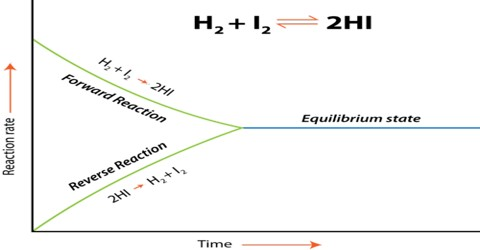# Calculations involving Chemical Equilibrium

Calculations involving Chemical Equilibrium

For solving most problems relating to finding equilibrium moles and equilibrium constant the following steps can be followed;

1. Set up a table of moles of each species on equilibrium stating initial (starting) moles, change of moles in terms of a, and equilibrium moles involving a.
2. If partial pressures are to be found, calculate the total moles (involving ‘a’) at equilibrium, and hence the mol fraction of each species.
3. For calculating Ke the values of the equilibrium concentrations in mol calculated from step 1 are to be substituted in the appropriate expression for Ke.
4. For calculating KP, the mol fraction of each species obtained in step 2 is multiplied by the equilibrium pressure to obtain values of partial pressures of the components in the mixture. Substitution of the partial pressure values in the appropriate expression for KP gives the result.